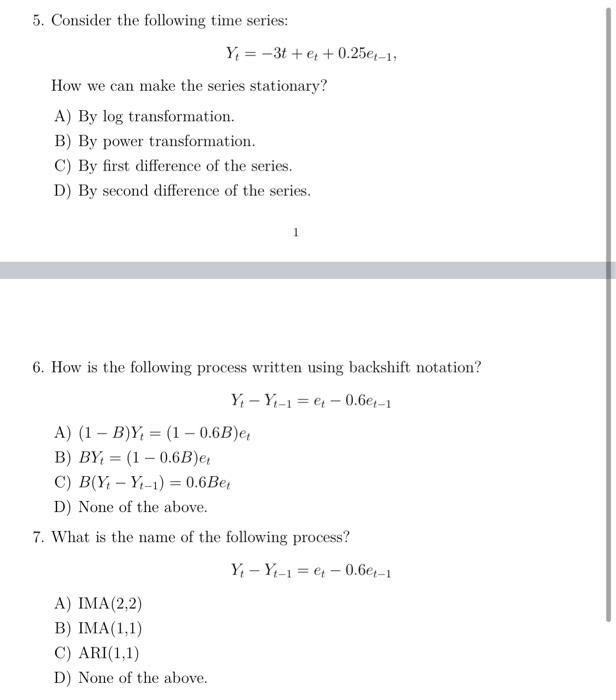# Question 5. Consider the following time series: Y = -3t + e + 0.25e-1, How we can make the series stationary? A) By log transformation. B) By power transformation. C) By first difference of the series. D) By second difference of the series. 6. How is the following process written using backshift notation? Y - Y-1 = e - 0.64-1 A) (1 - B)Y, = (1 -0.6B) B) BY = (1 -0.6B)e C) B(Y, -Y-1) = 0.6 Bei D) None of the above. 7. What is the name of the following process? Y, -Y-1 = e - 0.6-1 A) IMA(2,2) B) IMA(1,1) C) ARI(1,1) D) None of the above.